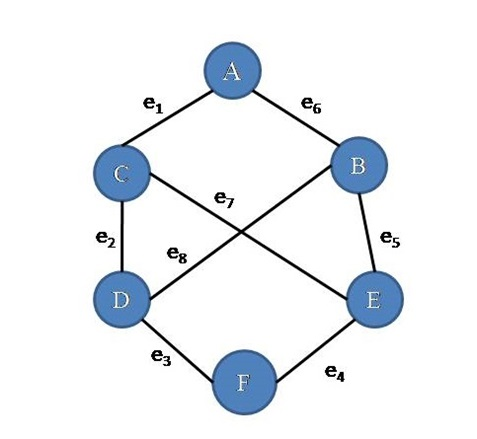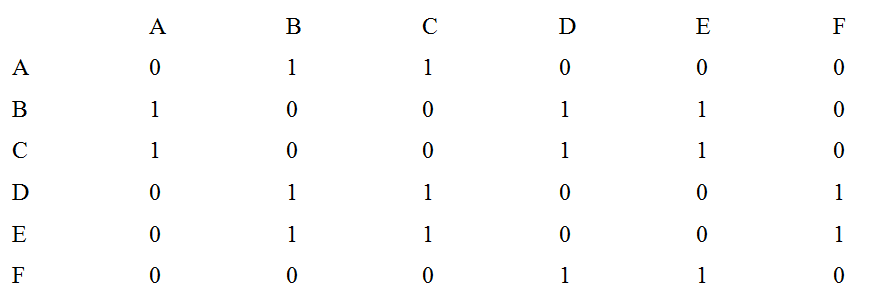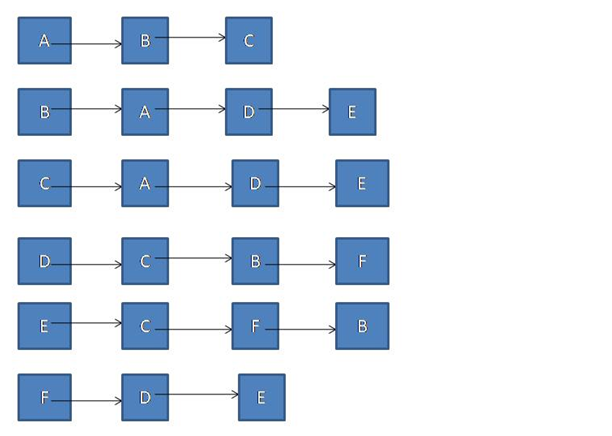# Representation of a Graph in Data Structure

Graph representation: In this article, we are going to see how to represent graphs in data structure?
Submitted by Souvik Saha, on March 17, 2019

What you will learn?

In this article we are going to study how graph is being represented?

Following is an undirected graph,We can represent the same graph by two different methods:

A graph can represent matrix elements. Initially, all the elements of a matrix are zero. If there is an edge between two vertices (example vertex A and B) then we mark '1' to the element at the position MAB and MBA for undirected graph and for a directed graph, we mark '1' to the element at the position MAB.

Example:A graph can also be represented by a list. We need an array of the list and for each time if there is an edge exist between two vertices then we push one vertex to another vertex list and vice versa. For the previous graph, we get an Adjacency List like this:Top MCQs